Khan and Koo: The Effect of Multiple Energy Detector on Evidence Theory Based Cooperative Spectrum Sensing Scheme for Cognitive Radio Networks

### Abstract

Spectrum sensing is an essential function that enables cognitive radio technology to explore spectral holes and resourcefully access them without any harmful interference to the licenses user. Spectrum sensing done by a single node is highly affected by fading and shadowing. Thus, to overcome this, cooperative spectrum sensing was introduced. Currently, the advancements in multiple antennas have given a new dimension to cognitive radio research. In this paper, we propose a multiple energy detector for cooperative spectrum sensing schemes based on the evidence theory. Also, we propose a reporting mechanism for multiple energy detectors. With our proposed system, we show that a multiple energy detector using a cooperative spectrum sensing scheme based on evidence theory increases the reliability of the system, which ultimately increases the spectrum sensing and reduces the reporting time. Also in simulation results, we show the probability of error for the proposed system. Our simulation results show that our proposed system outperforms the conventional energy detector system.

### 1. Introduction

A frequency spectrum is a limited natural resource, which is insufficient for increasing the number of wireless services. Thus, these resources should be properly utilized to improve the performance of the system [1,2]. According to the Federal Communication Commission (FCC) spectrum task force report, the usage of an allocated spectrum varies depending on temporal and geographical situations . The report says that the spectrum is unused by up to 85% in some cases. Consequently, the inefficient use, rather than actual physical scarcity, is the cause for insufficiency.
Cognitive radio (CR) is emerging as a tempting solution to the spectral congestion problem as it resourcefully accesses the frequency spectrum that is not occupied by the licensed user (LU). CR has the capability to adapt to its environment by changing its parameters, such as modulation, frequency, etc. . CR uses various techniques to utilize the unoccupied spectrum while guaranteeing the minimum interference level by the LU. The unoccupied spectrum is found by using the spectrum sensing technique, which is a key function of CR, to decide if the LU is present or absent. CR spectrum sensing, whose goal is to detect the presence or absence of the LU signal in the desired frequency band, is a challenging task due to the lack of cooperation from the LU. Thus, the way to how the spectrum band is handled is a crucial task for the spectrum sensing technique .
Data from multiple users can be combined either centrally or in a distributed manner and this data is combined at the fusion center (FC). If a single energy detector (currently used by CR) fails, then the CR cannot communicate, even though there is an opportunity for communication. It shows that the energy detector has a vital role in CR. Fading and shadowing also have a strong effect on the performance of wireless communication. Currently, the introduction of multiple antennas in the wireless communication field has given a new dimension to CR research. A multiple energy detector (MED) mitigates the fading and shadowing effects. MED takes advantage of multi-path fading to enhance the performance of the system. In , the authors proposed a semi-blind detection method for a cognitive radio network in multiple antenna systems. Similarly in , the author improved the spectrum sensing technique using multiple energy detectors for cognitive radio networks. However, all of these works considered traditional conventional decision rules.
In this paper, we focus on a MED in cooperative spectrum sensing based on the evidence theory in a Rayleigh fading channel. For our proposed system, we collected the results from MEDs at the CUs by using the equal gain combining (EGC) method. Instead of sending the local results randomly to the FC, CUs sent it according to their reliability. In our simulation results, we show that our proposed scheme outperforms the traditional cooperative spectrum sensing and reporting time. The contributions of this paper are as follows:
• We have proposed a MED in cooperative spectrum sensing based on the evidence theory in a Rayleigh fading channel.

• We have also proposed an ordered sequential reporting scheme, which is based on a contention-aware reporting mechanism. This mechanism ensures that a CU with high reliability will transmit the sensing result with priority.

• In our simulation results, we show that our proposed scheme outperforms the traditional cooperative spectrum sensing and reduce reporting time.

The remainder of this paper is organized as follows: Section 2 describes the related work provides and literature review. Section 3 explains our proposed multiple energy detector system model. In Section 4, we describe cooperative spectrum sensing based on the evidence theory for our proposed multiple energy detector. Section 5 explains the proposed reporting mechanism. In Section 6, the simulation results are shown and the paper is concluded in Section 7.

### 2. Related Work and Literature Review

In wireless communication, the quality of service (QOS) and reliability of data plays a vital role in system performance and should be considered as a priority . The main objective in wireless communication is to utilize the spectrum efficiently with minimum energy consumption and communication overhead . Different mechanisms are used to increase the system’s efficiency and performance .
In conventional cooperative spectrum sensing, each unlicensed user makes its local decision, which is sent to the FC for a final decision, various fusion rules can be used at the FC like AND, OR, Majority rule, etc. Improvement in performance can be achieved by using cooperative spectrum sensing over the single node sensing method . Unlike conventional sensing, the detection performance is significantly improved by a data fusion scheme that is based on the evidence theory without prior knowledge of the LU signal [14,15].
To overcome the limitation of a single energy detector, a MED has been proposed. A number of researchers have investigated multiple antennas from various points of view in various areas of research . The author in  intensively studied the performance of cooperative spectrum sensing and multiple antennas analytically. In , the author has quantified the detection performance as a function of antenna correlation in branches and the number of antennas and employed the square law combination. The optimal weight for each antenna is found to increase the performance of cooperative spectrum sensing . In , the author considered an overlay spectrum sharing scheme, which involves the antenna weight and power allocation for both the LU and SU, in order to enhance the performance of the cognitive radio networks. However, all of these works either considered conventional decision rules or a single energy detector. If the energy detector fails, the performance of the system is greatly degraded. In , the authors proposed a Bayesian approach for adaptive multiple antenna sensing in a cognitive radio network. This is capable of efficiently learning about the posterior distributions under both hypotheses, but this approach is specifically for multi antenna cognitive receivers. Our proposed scheme combines a MED with the D-S evidence theory, which results in high reliability in the uncertainty and performs more efficiently than the conventional single energy detector.

### 3. Proposed Multiple Energy Detector System Model

In order to protect LU from secondary user (SU) interference, spectrum sensing is performed by an SU to confirm whether the band is vacant or occupied. Various detection techniques, such as cyclo-stationary feature detection, matched filter detection, and energy detection have been used for LU detection. In this paper, we have been studied energy detection, which is easy to implement, and no prior knowledge of the LU is required.
The proposed MED system model is shown in Fig. 1. The model consists of an LU and a cognitive user (CU), which contain M number of energy detectors, and each energy detector consist of a single antenna. The resulting signal from all the antennas is combined using the EGC method at the CU.
Each CU performs local sensing by using MEDs. After local sensing, the information is transmitted in an ordered sequence of the sensing data reliability to the data fusion center (DFC). At the DFC, the final decision is made on the basis of the combined reported data.
The local spectrum sensing at each CU (having a single energy detector) can be formulated as a binary hypothesis as follows:
##### (1)
Xi={w(n):H0hi(n)s(n)+w(n):H1
where H0 and H1 are the absence and presence of the LU, respectively, Xi is the signal received at the ith CU, hi(n) is the fading channel coefficient, s(n) is the LU signal, and w(n) is the additive white Gaussian noise (AWGN).
In the case of MEDs, this local sensing can be formulated as:
##### (2)
Xi,j={wi,j(n):H0hi,j(n)s(n)+wi,j(n):H1
where i =1, 2,…, Nc is the number of CU, j = 1,2,…, M is the number of antennas, and n = 1,2,…, N is the number of samples. Xi.j is the received signal at the ith CU at the jth antenna, hi,j is the fading channel coefficient of the ith CU at the jth antenna, and wi,j(n) is the AWGN.
We assume that the MED is uncorrelated. CUs are independent and share the same spectrum with the LU. In a MED, the signal from each antenna is multiplied with the weighting factor ω.
Energy Ei,j of the received signal at the jth antenna of the ith CU is given as:
##### (3)
Ei,j=n=1Nωi,jXi,j(n)2
The energy detector Ei of each CU is given as:
##### (4)
Ei=j=1MEi,j
where Xi,j(n) is the sample received signal and N = 2TW, where T is the detection and W is the bandwidth.
The energy measured at each detector is combined using the EGC method, which is relatively easy to implement and has a low complexity as compared to other combination methods. In this paper, we utilized the EGC method to combine signals at the CU from the MED. The signal from MEDs can be combined at the CU by using the EGC method as:
##### (5)
E=i=1NcEi
When N is relatively large enough, E can be well approximated as a Gaussian random variable under both hypotheses H0 and H1, and it follows chi-square distribution with a degree of freedom MN . According to the central limit theorem (CLT) the detection problem in Eq. (2) can be written as:
##### (6)
{H0:μ0=MN,σ02=2MNH1:μ1=MN(γi,j+1),σ12=2MN(2γi,j+1)
where μ0, μ1 are the means and σ0 and σ1 are the variances of E under the hypotheses H0 and H1, respectively, and γi,j is the signal to noise ratio (SNR) of the primary signal at CU.

### 4. Evidence Theory Based Sequential Data Fusion for the Proposed Multiple Energy Detector

In this paper, we use the evidence theory for making final decision about the presence or absence of an LU at the DFC. In order to apply the evidence theory to cooperative spectrum sensing for deciding LU presence or absence, the discernment frame Fr can be defined as {H1, H0,Ω}, where Ω is the ignorance hypotheses, which describes that either of the hypotheses {H1, H0} is true. After the local sensing is carried out, which is done by the proposed MED, each CU estimates its self-assessed decision credibility, which is equivalent to the Basic Probability Assignment (BPA) for two hypotheses, as shown in Fig. 2. The BPA function is defined as a form of a cumulative density function as follows :
##### (7)
mi(H0)=Ei+12πσ0iexp(-(x-μ0i)22σ20i)dx
##### (8)
mi(H1)=-Ei12πσ1iexp(-(x-μ1i)22σ21i)dx
##### (9)
mi(Ω)=1-mi(H0)-mi(H1)
where mi(H0), mi(H1), and mi(Ω) are the BPA hypothesis of the ith CU, respectively. These values are sent to the DFC by CUs, and the DFC makes a global decision about the presence or absence of a LU.
According to the evidence theory, the BPA can be combined as :
##### (10)
m(H0)=m1(H0)m2(H0)mi(H0)=Fr1Fr2Frn=H0i=1nmi(Fri)(1-k)
##### (11)
m(H1)=m1(H1)m2(H1)mi(H1)=Fr1Fr2Frn=H1i=1nmi(Fri)(1-k)
where k=Fr1Fr2Frn=i=1nmi(Fri) is the amount of conflict between mass sets, and Fri is the ith element of the set {H1, H0, Ω} given in the frame of discernment and mi(Fri) is the BPA of ith element. The ⊕ also denotes the combination operator denoted by Eq. (14).
A simple decision strategy is chosen for the global decision. The main problem in the evidence-based data fusion process is that a large number of communication resources for reporting the sensing results are required. In order to reduce these communication overheads, processing time, and energy consumption for sensing, we consider using sequential cooperative spectrum scheme. In this scheme, after local sensing performed by CU, each CU transmits the sensing data to the DFC on the basis of its reliability, which is given as:
##### (12)
ri=|logmi(H1)mi(H0)|
The sensing data at the DFC are then combined using the evidence theory. At the DFC, the BPA is sequentially combined in the order that the data arrives as:
##### (13)
mk,global(Hj)=mk-1,global(Hj)mk(Hj)
Where j = [0,1], mk,global(Hj), mk−1,global(Hj), and are the kth and (k − 1)th global BPA hypothesis, Hj, respectively, and the combination operator ⊕ is defined on the basis of the evidence theory as follows:
##### (14)
ma(Hj)mb(Hj)=ma(Hj)mb(Ω)+ma(Hj)mb(Hj)+ma(Ω)mb(Hj)1-[(ma(Hj)mb(H1-j)+ma(H1-j)mb(Hj)]
##### (15)
ma(Ω)mb(Ω)=1-mamb(H1)-mamb(H0)
where j = [0,1] and a and b denote the two arbitrary combining sources.
The evidence theory global decision will be based on the global combination ratio rk,global of the k reports available at the DFC, which is defined as:
##### (16)
rk,global=log[mk,global(H1)mk,global(H0)]
The proposed scheme follows strategies for making the final decision.
1. In the first case, when the number of reports (k) available at DFC is less than the total number of CUs (Nc) (i.e., (k < Nc )) the global decision Dglobal can be calculated as:

##### (17)
Dglobal={H0;Ifrk,global<-λH1;Ifrk,global>λnodecision;If-λ<rk,global<λ
where −λ< rk,global < λ, represents that the reports at the DFC are not enough to make a decision, and waits for the next data report.
2. In the second case, when the total number of reports (k) available at the DFC is equal to the number of CUs (i.e., (k = Nc)), the global decision Dglobal can be measured as:

##### (18)
Dglobal={H0Ifrk,global<0H1Ifrk,global>0
In the next section, we discuss the reporting method for the proposed method.

### 5. Proposed Reporting Method

In this section, we present the description of the proposed reporting method. The reporting method helps to reduce the fusion time due to the combination efficiency of highly reliable data. The decision fusion process for determining the spectrum availability or occupancy has two steps.
In the first step, each CU senses the spectrum locally. In the second step, each CU tries to send its sensed data to the DFC in an ordered sequential manner, which relies on the CU’s self-assessed credibility ratio (ri), which was explained in the previous section. Since all the CUs share a common channel, this results in a collision. To avoid a collision and improve performance, different algorithms have been proposed.
In the reporting period, the DFC defines a data reliability range. If the CU sensed data is in this range, the CU decides to transmit its data; otherwise it remains silent. Each transmission made during the reporting period is preceded by a contention slot in which the CUs contend for access to the channel. If the CU does not sense any activity on the channel for a period of time toffset, it wins the contention slot and transmits a burst signal, followed by transmitting the local decision data to the DFC. Since CUs sense the channel for availability and try to avoid any collisions, this mechanism can be referred to as a contention-aware reporting method, as shown in Fig. 3.
The period of the node’s contention is defined as the contention slot, which should be performed before every reporting slot.
In the kth contention slot, the value of the offset toffset is calculated as follows:
##### (19)
toffset=tcon-2tslot(rk,max-rk,min)(rk,max-ri)+tslot
where tslot is the slot time corresponding to the time required by the radio link layer for carrier sensing and tcon is the length of the contention. rk,max and rk,min are the maximum and minimum values of the data sensing reliability at the kth contention slot, respectively. The value of rk,max and rk,min are first time defined by the DFC, and are then updated automatically after every reporting slot.
Whenever the DFC makes a global decision, it generates a burst signal in all the lengths of contention slots for obtaining a reservation for transmitting in the next reporting slot.
The reporting time Treport of the contention-aware reporting method can be calculated as:
##### (20)
Treport=Nc×Pcon×(treport+tcon)
where Pcon is the percentage of CUs contending for access to the channel because their sensed data falls in the reliability range defined by the DFC. tcon and treport are the times for a single contention and reporting slot, respectively. Nc is the total number of CU nodes.
In the next section, we present the simulation results and analysis for the proposed system.

### 6. Simulation Results and Analysis

In this section, we present the simulation results of our proposed MED systems based on the evidence theory and compare their performance with the conventional energy detector employing ordered sequential fusion. We carried out our simulation by using MATLAB, and the simulation parameters are defined in Table 1.
For our simulation, we used one LU and 100 SU nodes. We also considered the effect on the system by changing the number of antennas. We varied SNRs in the range of [−22 to −18], while the value of λ varied from 0 to 30. We evaluated the performances of the proposed scheme in terms of tested the probability of detection, the probability of false alarm, the probability of error, the reporting number percentage, and the average reporting time. We then compared these performances with those of the conventional DS energy detector.
In Fig. 4, we have shown the performance comparison for the probability of detection between the conventional DS energy detector and the proposed multiple energy detector for various SNR values. It can be observed that, with the increase of the SNR value the probability of detection increases, which is an indication that the reliability of the system is increasing. It is also shown in Fig. 4 that the performance of the proposed MED is better than the conventional DS energy detector. Specifically, the probability of detecting a conventional DS energy detector at −18 dB is just above 0.9, while in the case of the proposed MED scheme, we achieved a detection probability of approximately 0.92 at a lower SNR than the conventional scheme (i.e., at SNR = −20 dB). This result shows the effectiveness of the proposed MED scheme, which is a clear indication of the advantage of the proposed MED scheme over single or conventional DS energy detectors.
In Fig. 5, we illustrate the performance of the probability of a false alarm for the proposed MED and conventional DS energy detector for various SNR values. As seen in Fig. 5, the performance of the proposed MED is better than the conventional DS energy detector. For example, the probability of a false alarm of the conventional DS energy detector is less than 0.1 at −18 dB, while we get a much lower probability of a false alarm at SNR = −20 dB in the case of the proposed MED scheme. Thus, we can conclude that the proposed system has better performance than the conventional DS energy detector.
Similarly, Fig. 6 shows the simulation results for the probability of detection and the probability of a false alarm for a conventional DS energy detector and the proposed MED for various SNR values. For simulation in Fig. 6, we changed the number of antenna from three to five. The objective of changing the number of energy detectors was to show the importance of the proposed method in terms of the probability of detection and the probability of a false alarm. It can be observed in Fig. 6 that the probability of detection increases and the probability of a false alarm decreases with the increase in the number of antennas. Also, it is worth mentioning here that with an increase in the number of energy detectors, the reliability of the system increases, which takes advantage of multi-path fading. The simulation results show that our proposed MED scheme outperforms the single or conventional DS energy detector schemes.
In Fig. 7, we show the probability of detection at SNR= −20 dB, and we changed the number of energy detectors. Here the importance of the energy detector can be observed (i.e., with the increase in the number of energy detectors, the detection probability of the systems increase, but on the other hand, the complexity of the system also increases).
Fig. 8 shows the simulation result for the percentage of the reporting number vs. the threshold value for the proposed MED and conventional DS energy detector. The proposed sequential fusion is more effective for reducing percentage of reporting number as compared to the conventional fusion scheme. It is demonstrated in the simulation that the percentage of reporting number of the proposed scheme is less than the conventional DS energy detector. Specifically, at SNR = −18 dB, when the threshold value is 25, the reporting number percentage of the proposed MED is 23%; while for the conventional DS energy detector it is 58%. It can be observed that the conventional scheme requires more than twice the reporting number, as that of the proposed scheme. This shows that the conventional scheme requires more information than the proposed method for obtaining higher accuracy. The proposed system converges at a lower reporting number than the conventional DS energy detector, and it reduces the communication overhead.
Fig. 9 shows the average reporting time vs. the number of nodes in the network. It can be seen that the reporting time decreases as the number of nodes in the network increases. The reason for this is that the order of the sequential fusion provides better utilization of the sensing data diversity as the number of nodes increases. Thus, it can be seen that our proposed MED systems takes advantage of diversity and produces better results than those of the conventional DS energy detector.
In Fig. 10, we have shown the probability of error comparison for the proposed MED and conventional DS energy detector scheme for various SNRs (i.e., [−22 −20 −18], when MED=5). The probability of error decreases with the increase in SNR. As the SNR increases, the probability of detection increases and the probability of miss-detection and false alarm decreases, which ultimately increases the reliability of the system and decreases the probability of error. It can be observed when the SNR increases from −22 to −18 dB, the probability of error decreases, which shows the effectiveness of the proposed method. For example, the probability of error for the conventional DS energy detector is 0.3 at the threshold value of λ =10. However, with the same parameter we achieve a 0.1 probability of error for the proposed scheme, which shows that the proposed scheme is more effective than the conventional DS energy detector. Thus, we can conclude from the simulation results that the proposed MED scheme outperforms the single or conventional DS energy detector schemes.

### 7. Conclusion

In this paper, we have proposed a MED in a cooperative spectrum sensing based on the evidence theory. We have shown that the performance of the proposed MED is better than a conventional DS energy detector. We have shown in the simulation results that the proposed MED is more reliable. The probability of detection is high, as compared to a conventional DS energy detector at a low SNR. Similarly, the probability of a false alarm of the proposed MED is lower than that of the conventional DS energy detector. We have also shown in the simulation that the percentage of reporting number and the average reporting time of the proposed MED is less than the conventional energy detector. Furthermore, the probability error result validates the performance of our proposed scheme. Thus, our proposed MED scheme outperforms the conventional DS energy detector system.

### Acknowledgement

This work was supported by the National Research Foundation (NRF) of Korea funded by the MEST (Nos. NRF-2015R1D1A1A09057077 and NRF-2015R1A2A1A15053452).

### Biography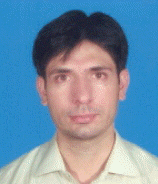He received the B.Sc. degrees in Computer Information Systems Engineering from UET Peshawar, Pakistan in 2004 and his M.Eng in Telecom & Control Engineering from MUET Jamshoro, Pakistan in 2007. Currently he is pursuing his doctorate degree as a member of the multimedia communication system lab at University of Ulsan. His research interests includes Cognitive radio, Cooperative communication network, Wireless sensor networks.

### Biography

Insoo Koo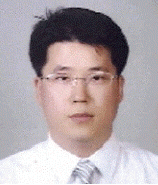He received his B.E. from Konkuk University, Seoul, Korea, in 1996, and his M.S and Ph.D. degree from Gwangju Institute of Science and Technology (GIST) Gwangju, Korea in 1998 and 2002, respectively. From 2002 to 2004, he worked with Ultrafast Fiber-Optic Networks (UFON) Research Center in GIST as a research professor. For one year from September 2003, he was a visiting scholar at Royal Institute of Science and Technology, Sweden. In 2005, he joined University of Ulsan where he is now a professor. His research interests include next-generation wireless communication systems and wireless sensor networks.

### References

1. SP. Algur, and NP. Kumar, "Novel user centric, game theory based bandwidth allocation mechanism in WiMAX," Human-Centric Computing and Information Sciences, vol. 3, no. 20, pp. 1-20, 2013.2. GHS. Carvalho, I. Woungang, A. Anpalagan, and SK. Dhurandher, "Energy-efficient radio resource management scheme for heterogeneous wireless networks: a queueing theory perspective," Journal of Convergence, vol. 3, no. 4, pp. 15-22, 2012.

3. Federal Communications Commission, in Notice of proposed rule making and order, Federal Communications Commission, Washington, DC: FCC 03-322, 2003.

4. A. Gorcin, and B. Thiagarajan, "A signal identification application for cognitive radio," in Proceedings of SDR Forum Technical Conference, Denver, CO, 2007.

5. U. Habiba, Md I. Islam, and MR. Amin, "Performance evaluation of the VoIP services of the cognitive radio system, based on DTMC," Journal of Information Processing Systems, vol. 10, no. 1, pp. 119-131, 2014.6. R. Ujjinimatad, and SR. Patil, "Semi-blind detection method for cognitive radio networks in multiple antenna systems," in Proceedings of 2013 Annual International Conference on Emerging Research Areas and 2013 International Conference on Microelectronics, Communications and Renewable Energy (AICERA/ICMiCR), Kanjirapally, India, 2013, pp. 1-6.7. A. Bagwari, and GS. Tomar, "Improved spectrum sensing technique using multiple energy detectors for cognitive radio networks," International Journal of Computer Applications, vol. 62, no. 4, pp. 11-21, 2013.8. H. Elshaafi, and D. Botvich, "Trustworthiness inference of multi-tenant component services in service compositions," Journal of Convergence, vol. 4, no. 1, pp. 31-37, 2013.9. A. Sinha, and DK. Lobiyal, "Performance evaluation of data aggregation for cluster-based wireless sensor network," Human-Centric Computing and Information Sciences, vol. 3, no. 1, pp. 1-17, 2013.10. M. Yoon, YK. Kim, and JW. Chang, "An energy-efficient routing protocol using message success rate in wireless sensor networks," Journal of Convergence, vol. 4, no. 1, pp. 15-22, 2013.

11. SK. Bae, "Power consumption analysis of prominent time synchronization protocols for wireless sensor networks," Journal of Information Processing Systems, vol. 10, no. 2, pp. 300-313, 2014.12. SMA. Zaidi, and B. Song, "Prioritized multipath video forwarding in WSN," Journal of Information Processing Systems, vol. 10, no. 2, pp. 176-192, 2014.13. N. Nguyen-Thanh, and I. Koo, "An enhanced cooperative spectrum sensing scheme based on evidence theory and reliability source evaluation in cognitive radio context," IEEE Communications Letters, vol. 13, no. 7, pp. 492-494, 2009.14. Q. Peng, K. Zeng, J. Wang, and S. Li, "A distributed spectrum sensing scheme based on credibility and evidence theory in cognitive radio context," in Proceedings of 2006 IEEE 17th International Symposium on Personal, Indoor and Mobile Radio Communications, Helsinki, Finland, 2006, pp. 1-5.

15. N. Nguyen-Thanh, and I. Koo, "An efficient ordered sequential cooperative spectrum sensing scheme based on evidence theory in cognitive radio," IEICE Transactions on Communications, vol. 93B, no. 12, pp. 3248-3257, 2010.16. JH. Chong, CK. Ng, NK. Noordin, and BM. Ali, "A low computational complexity V-BLAST/STBC detection mechanism in MIMO system," Human-centric Computing and Information Sciences, vol. 4, no. 1, pp. 1-28, 2014.

17. JH. Chong, CK. Ng, NK. Noordin, and BM. Ali, "Dynamic transmit antenna shuffling scheme for MIMO wireless communication systems," Journal of Convergence, vol. 4, no. 1, pp. 7-14, 2013.18. RF. Ustok, and B. Ozbek, "Spectrum sensing based on energy detection for cognitive radio systems with multiple antennas," in Proceedings of IEEE 17th Signal Processing and Communications Applications Conference (SIU2009), Antalya, Turkey, 2009, pp. 396-399.19. A. Salarvan, and GK. Kurt, "Multi-antenna spectrum sensing for cognitive radio under Rayleigh channel," in Proceedings of 2012 IEEE Symposium on Computers and Communications (ISCC), Cappadocia, Turkey, 2012, pp. 780-784.20. H. Wang, G. Noh, D. Kim, S. Kim, and D. Hong, "Advanced sensing techniques of energy detection in cognitive radios," Journal of Communications and Networks, vol. 12, no. 1, pp. 19-29, 2010.21. VRS. Banjade, N. Rajatheva, and C. Tellambura, "Performance analysis of energy detection with multiple correlated antenna cognitive radio in Nakagami-m fading," IEEE Communications Letters, vol. 16, no. 4, pp. 502-505, 2012.22. Y. Ou, and YM. Wang, "Multiple antennas spectrum sensing for cognitive radio networks," Journal of Networks, vol. 8, no. 3, pp. 665-671, 2013.23. R. Manna, RH. Louie, Y. Li, and B. Vucetic, "Cooperative spectrum sharing in cognitive radio networks with multiple antennas," IEEE Transactions on Signal Processing, vol. 59, no. 11, pp. 5509-5522, 2011.24. J. Manco-Vasquez, M. Lazaro-Gredilla, D. Ramirez, J. Via, and I. Santamaria, "A Bayesian approach for adaptive multiantenna sensing in cognitive radio networks," Signal Processing, vol. 96B, pp. 228-240, 2014.25. W. Zhao, T. Fang, and Y. Jiang, "Data fusion using improved Dempster-Shafer evidence theory for vehicle detection," in Proceedings of 4th International Conference on Fuzzy Systems and Knowledge Discovery (FSKD2007), Haikou, China, 2007, pp. 487-491.##### Fig. 1
Proposed multiple energy detector (MED). LU=licenses user, CU=cognitive user.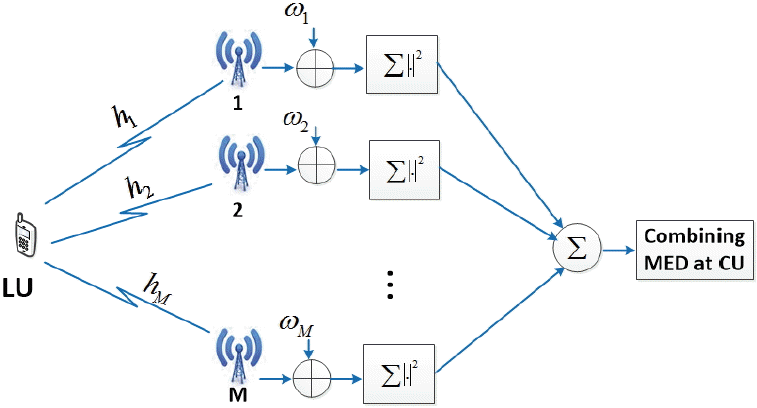##### Fig. 2
Multiple energy detector (MED) cooperative spectrum sensing scheme. LU=licenses user, CU= cognitive user.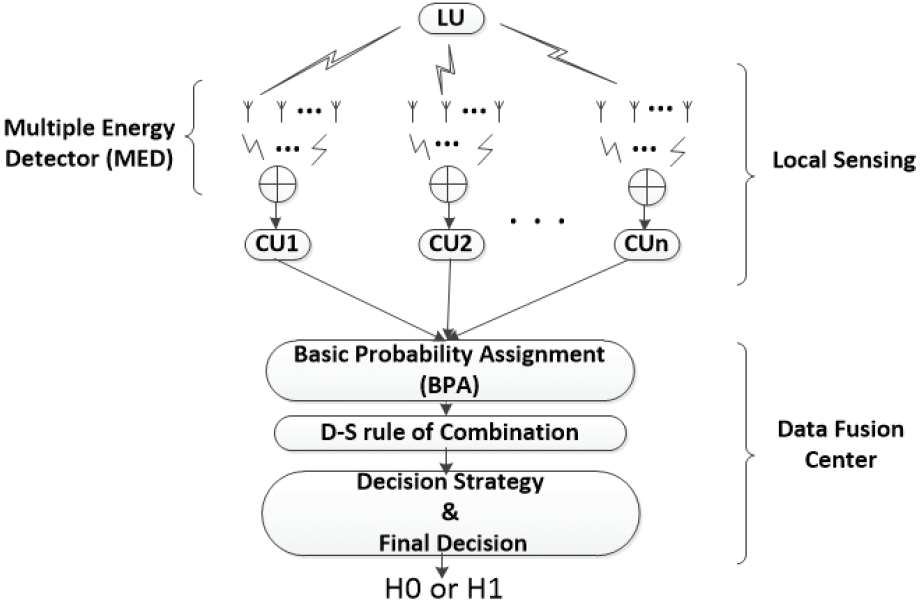##### Fig. 3
Reporting mechanism window for proposed multiple energy detector.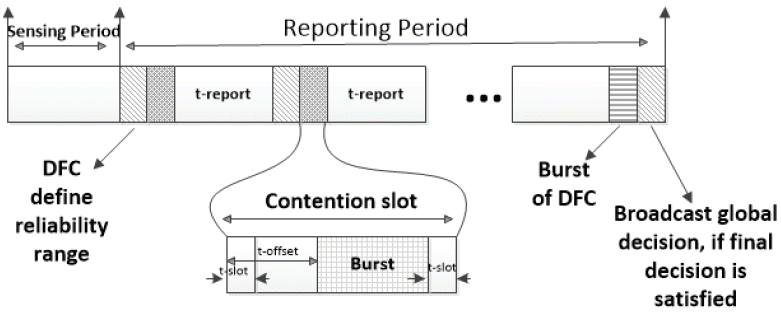##### Fig. 4
Probability of detection vs.λ, when multiple energy detector (MED) is 3. SNR=signal to noise ratio.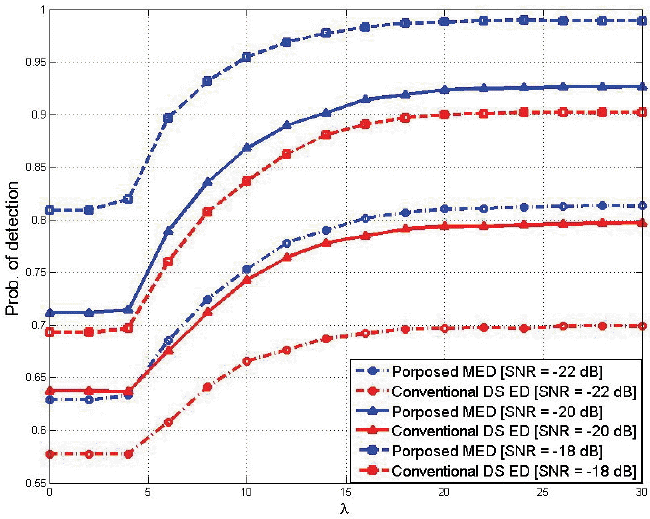##### Fig. 5
Probability of false alarm vs.λ, when multiple energy detector (MED) is 3. SNR=signal to noise ratio.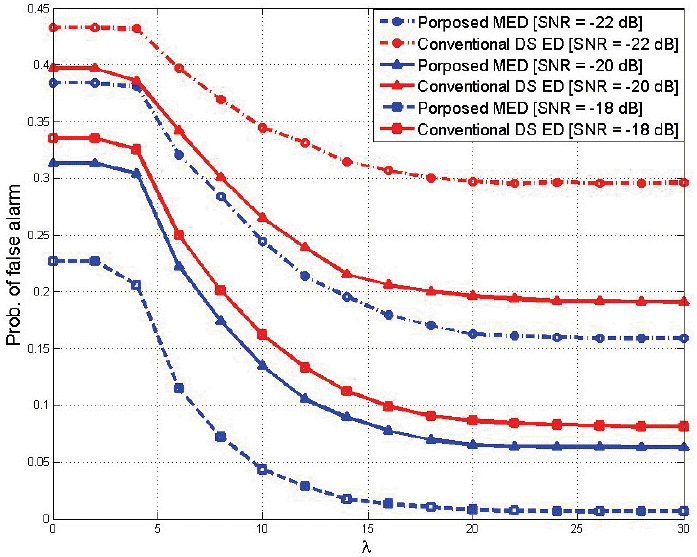##### Fig. 6
Probability of detection & false alarm vs. thresholdλ, when multiple energy detector (MED) is 5. SNR=signal to noise ratio.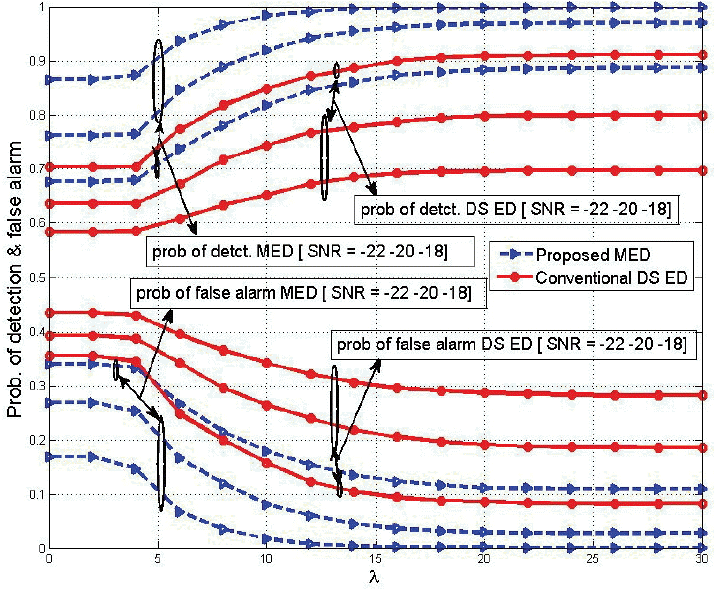##### Fig. 7
Probability of detection vs. λ, when number of multiple energy detector (MED) is 1, 3, and 5.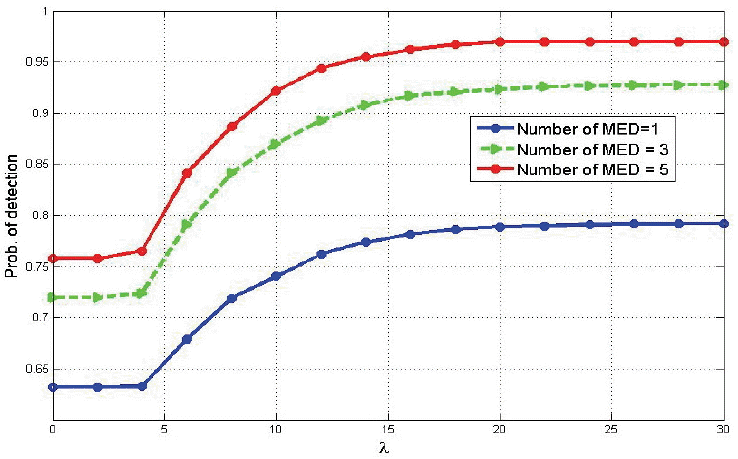##### Fig. 8
Percentage of reporting number vs.λ, when multiple energy detector (MED) is 5. SNR=signal to noise ratio.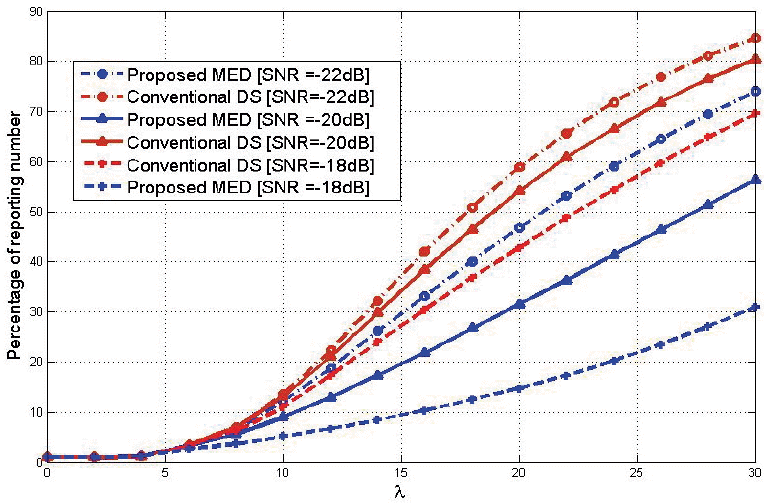##### Fig. 9
Average reporting time vs. number of nodes, when multiple energy detector (MED) is 5. SNR= signal to noise ratio.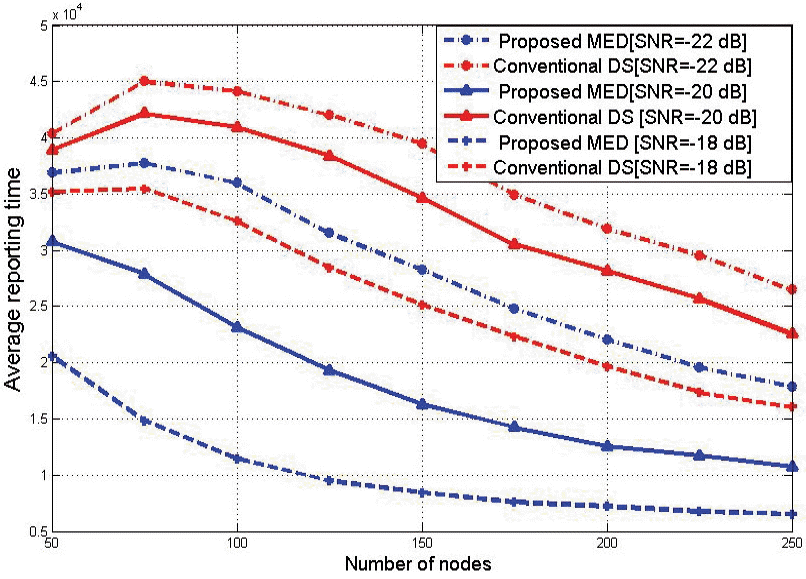##### Fig. 10
Probability of error, when multiple energy detector (MED) is 5. SNR=signal to noise ratio.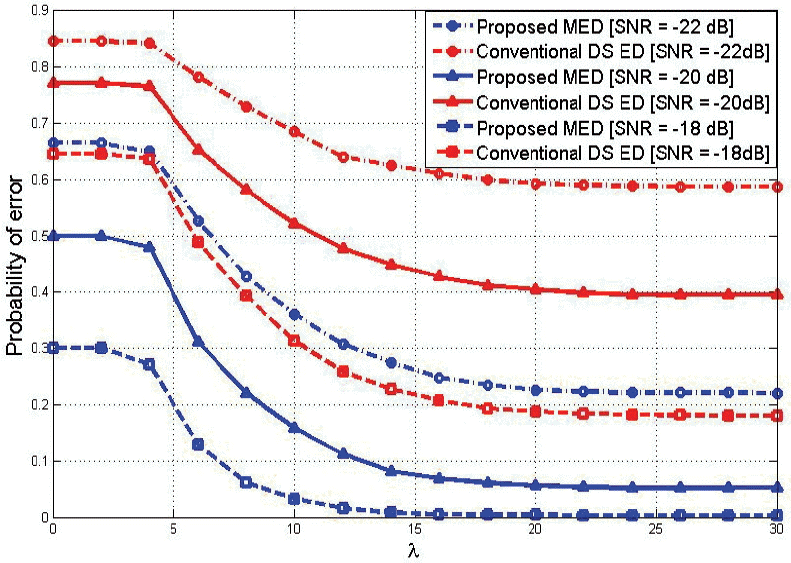##### Table 1
Simulation parameters
Parameter Value